## RS Aggarwal Class 8 Solutions Chapter 25 Graphs Ex 25C

These Solutions are part of RS Aggarwal Solutions Class 8. Here we have given RS Aggarwal Solutions Class 8 Chapter 25 Graphs Ex 25C.

Other Exercises

OBJECTIVE QUESTIONS
Tick the correct answer in each of the following:

Question 1.
Solution:
∵ In point (3, 6), both x and y are positive.

Question 2.
Solution:
∵ In point ( – 7, – 1) both x and y are negative.

Question 3.
Solution:
∵ In point (2, – 3), x is positive and y is negative.

Question 4.
Solution:
∵ In point ( – 4, 1), x is negative and y is positive.

Question 5.
Solution:
∵ Abscissa is distance of a point from y- axis

Question 6.
Solution:
y = a is a line parallel to x-axis at a distance of ‘a’ units.

Question 7.
Solution:
The equation of the line y-axis, is x = 0

Hope given RS Aggarwal Solutions Class 8 Chapter 25 Graphs Ex 25C are helpful to complete your math homework.

If you have any doubts, please comment below. Learn Insta try to provide online math tutoring for you.

## RS Aggarwal Class 8 Solutions Chapter 25 Graphs Ex 25B

These Solutions are part of RS Aggarwal Solutions Class 8. Here we have given RS Aggarwal Solutions Class 8 Chapter 25 Graphs Ex 25B.

Other Exercises

Question 1.
Solution:
(a) y = 3x
By giving some different values to x, we shall get corresponding values of y.
x = 1 then y = 3 x 1 = 3
if x = 2, then y = 3 x 2 = 6
if x = 0, then y = 3 x 0 = 0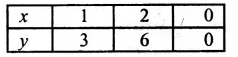Now plotting the points given above, and joining them.
(b) We get a line, From the graph.
(i) When x = 3, then y = 9
(ii) When x = 5, then y = 15
(iii) When x = 6, then y = 18 Ans.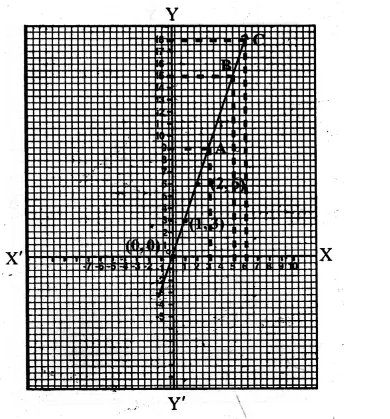Question 2.
Solution:
(a) P = 4x
By giving some different values to x, we get the corresponding values of y or P
If x = 1, then P = 4 x 1 = 4
if x = 2, then P = 4 x 2 = 8
if x = 0, then P = 4 x 0 = 0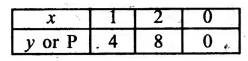Plot the points (1, 4), (2, 8) and 0, 0) on the graph and join then to get the graph of P = 4x as shown
(b) From the graph we see that
(i) When x = 3, then P = 12
(ii) When x = 4, then P = 16
(iii) When x = 6, then P = 24 Ans.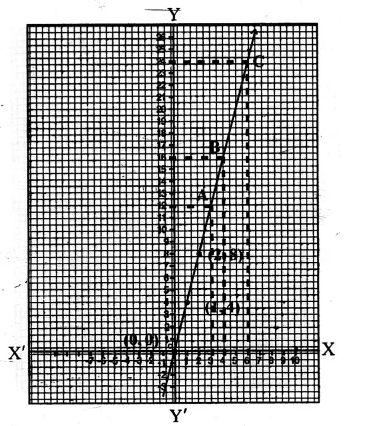Question 3.
Solution:
A = x²
giving some values to x, we get corresponding values of y or A
If x = 1, then y or A = (1)² = 1
If x = 2, then y or A = (2)² = 4
If x = 0, then y or A = (0)² = 0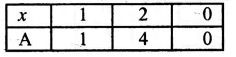Now plot the point (1, 1), (2, 4), (0, 0) on the graph, and join them to get the graph of A = x2 as shown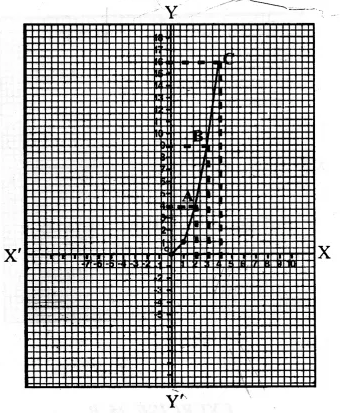(b) From the graph we see that
(i) When x = 2, then A = 4
(ii) When x = 3, then A = 9
(ii) When x = 4 then A = 16 Ans.

Hope given RS Aggarwal Solutions Class 8 Chapter 25 Graphs Ex 25B are helpful to complete your math homework.

If you have any doubts, please comment below. Learn Insta try to provide online math tutoring for you.

## RS Aggarwal Class 8 Solutions Chapter 25 Graphs Ex 25A

These Solutions are part of RS Aggarwal Solutions Class 8. Here we have given RS Aggarwal Solutions Class 8 Chapter 25 Graphs Ex 25A.

Other Exercises

Question 1.
Solution:
Below is given the graph in which X’OX and YOY’ are the co-ordinate axes intersecting each other at O. Now the. points given have been plotted as shown on the graph.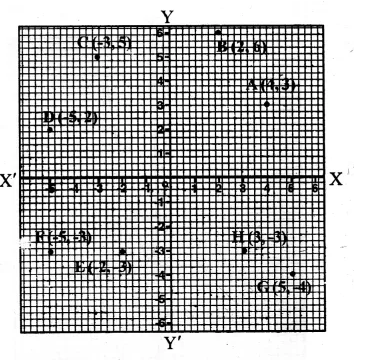Hope given RS Aggarwal Solutions Class 8 Chapter 25 Graphs Ex 25A are helpful to complete your math homework.

If you have any doubts, please comment below. Learn Insta try to provide online math tutoring for you.

## RS Aggarwal Class 8 Solutions Chapter 24 Probability Ex 24B

These Solutions are part of RS Aggarwal Solutions Class 8. Here we have given RS Aggarwal Solutions Class 8 Chapter 24 Probability Ex 24B.

Other Exercises

Tick the correct answer in each of the following :

Question 1.
Solution:
A spinning wheel has 3 white and 5 green sectors
Possible out come = 3 + 5 = 8
It is spinners, then
Probability of getting a green sector = $$\\ \frac { 5 }{ 8 }$$ (b)

Question 2.
Solution:
8 cards are numbered 1, 2, 3, 4, 5, 6, 7, 8
They are mixed and kept in a box One card is chosen at random, then Probability of card having 9 number less than 4 = $$\\ \frac { 3 }{ 8 }$$ (c)

Question 3.
Solution:
Two coins are tossed simultaneously, then Possible outcomes = 4
Now probability of getting one head and one tail = $$\\ \frac { 2 }{ 4 }$$ = $$\\ \frac { 1 }{ 2 }$$ (b)

Question 4.
Solution:
. In a bag, there are 3 white and 2 red balls
Possible outcomes = 3 + 2 = 5
Now probability of a red ball drawn
= $$\\ \frac { 2 }{ 5 }$$ (d)

Question 5.
Solution:
A die is thrown then
Possible outcomes = 6
Now probability of getting 6 is $$\\ \frac { 1 }{ 6 }$$ (b)

Question 6.
Solution:
A die is thrown
Possible outcomes = 6
Now probability of getting an even number
which are 2, 4, 6 = $$\\ \frac { 3 }{ 6 }$$ = $$\\ \frac { 1 }{ 2 }$$ (a)

Question 7.
Solution:
One card is drawn from a well shuffled deck of 52 cards, possible out comes = 52
The probability of card which is a queen = $$\\ \frac { 4 }{ 52 }$$
= $$\\ \frac { 1 }{ 13 }$$ (c)

Question 8.
Solution:
One card is drawn from a well-shuffled deck of 52 card, possible out comes = 52 Probability of a card being a black 6
(which are two) = $$\\ \frac { 2 }{ 52 }$$ = $$\\ \frac { 1 }{ 26 }$$ (b)

Hope given RS Aggarwal Solutions Class 8 Chapter 24 Probability Ex 24B are helpful to complete your math homework.

If you have any doubts, please comment below. Learn Insta try to provide online math tutoring for you.

## RS Aggarwal Class 8 Solutions Chapter 24 Probability Ex 24A

These Solutions are part of RS Aggarwal Solutions Class 8. Here we have given RS Aggarwal Solutions Class 8 Chapter 24 Probability Ex 24A.

Other Exercises

Question 1.
Solution:
(i) When a coin is tossed, we get outcomes 2 as H or T (Head or Tail)
(ii) When two coins are tossed together, we get possible four outcomes as HH, HT, TH, TT
(iii) A die is thrown, we get possible outcomes as 1,2, 3, 4, 5, 6
(iv) From a well – shuffled deck of 52 cards, 0ne card is at random drawn, we get the possible outcomes is 52

Question 2.
Solution:
Possible outcomes = 2
In a single throw of a coin, we get
probability of getting a tail = $$\\ \frac { 1 }{ 2 }$$

Question 3.
Solution:
In a single throw of two coins, possible outcomes = 4
(i) Probability of getting both tails = $$\\ \frac { 1 }{ 4 }$$
(ii) Probability of getting at least one tail = $$\\ \frac { 3 }{ 4 }$$
(iii) Probability of getting at the most one tail = $$\\ \frac { 2 }{ 4 }$$ = $$\\ \frac { 1 }{ 2 }$$

Question 4.
Solution:
In a bag, there are 4 white and 5 blue balls ,
Possible outcomes = 4 + 5 = 9
One ball is drawn at random, then
(i) the probability of a white ball = $$\\ \frac { 4 }{ 9 }$$
(ii) the probability of a blue ball = $$\\ \frac { 5 }{ 9 }$$

Question 5.
Solution:
In a bag, there are 5 white, 6 red and 4 green balls
Possible outcome is 5 + 6 + 4 = 15
One ball is drawn at random, then
(i) Probability of a green ball = $$\\ \frac { 4 }{ 15 }$$
(ii) Probability of a white ball = $$\\ \frac { 5 }{ 15 }$$ = $$\\ \frac { 1 }{ 3 }$$
(iii) Probability of a non-red ball = $$\\ \frac { 5+4 }{ 15 }$$
= $$\\ \frac { 9 }{ 15 }$$
= $$\\ \frac { 3 }{ 5 }$$
(5 white and 4 green balls are non-red balls)

Question 6.
Solution:
In a lottery, there are 10 prizes and 20 blanks
Possible outcomes = 10 + 20 = 30
A ticket is chosen at random, then
probability of getting a prize = $$\\ \frac { 10 }{ 30 }$$ = $$\\ \frac { 1 }{ 3 }$$

Question 7.
Solution:
In a ,box of 100 electric bulb, 8 are defective
Then non-defective bulbs = 100 – 8 = 92
Now possible outcomes = 100
(i) Probability of a drawn bulb, which is defective = $$\\ \frac { 8 }{ 100 }$$ = $$\\ \frac { 2 }{ 25 }$$
(ii) Probability of a drawn bulb which is non defective = $$\\ \frac { 92 }{ 100 }$$ = $$\\ \frac { 23 }{ 25 }$$

Question 8.
Solution:
A die is thrown, then
Possible outcomes = 6
(i) Now probability of getting 2 = $$\\ \frac { 1 }{ 6 }$$
(ii) Probability of a number less than 3 (which are 1 and 2) = $$\\ \frac { 2 }{ 6 }$$ = $$\\ \frac { 1 }{ 3 }$$
(iii) Probability of a composite number (a composite number is a number which is not a prime number which are 4, 6) = $$\\ \frac { 2 }{ 6 }$$ = $$\\ \frac { 1 }{ 3 }$$
(iv) Probability of a number not less than 4 (which are 5, 6) = $$\\ \frac { 2 }{ 6 }$$ = $$\\ \frac { 1 }{ 3 }$$

Question 9.
Solution:
Total number of ladies = 200
Those who like coffee = 82
Those who dislike coffee = 118
Possible number of outcomes = 200
One lady is chosen at random, then
(i) Probability of a lady who dislikes coffee = $$\\ \frac { 118 }{ 200 }$$
= $$\\ \frac { 59 }{ 100 }$$

Question 10.
Solution:
19 ball bearing numbers, 1, 2, 3,…19
possible outcomes = 19
A ball is drawn at random from the box, then
(i) Probability of a ball which bears a prime numbers which are 2, 3, 5, 7, 11, 13, 17 and 19 = 8 = $$\\ \frac { 8 }{ 19 }$$
(ii) Probability of a ball which bears an even number which are 2, 4, 6, 8, 10, 12, 14, 16, 18 = 9 = $$\\ \frac { 9 }{ 19 }$$
(iii) Probability of a number which bears a number divisible by 3 which are 3, 6, 9, 12, 15, 18 = 6 = $$\\ \frac { 6 }{ 19 }$$

Question 11.
Solution:
A card’s drawn at random from a deck
of well-shuffled deck of 52 cards Probability = 52
(i) Probability of a card being a king = $$\\ \frac { 4 }{ 52 }$$ = $$\\ \frac { 1 }{ 13 }$$
(ii) Probability of a card being spade = $$\\ \frac { 13 }{ 52 }$$ = $$\\ \frac { 1 }{ 4 }$$
(iii) Probability of a card being a red queen = $$\\ \frac { 2 }{ 52 }$$ = $$\\ \frac { 1 }{ 26 }$$
(iv) Probability of a card being a black 8 = $$\\ \frac { 2 }{ 52 }$$ = $$\\ \frac { 1 }{ 26 }$$

Question 12.
Solution:
One card is drawn at random from a deck of well shuffled deck of 52 cards
Possible outcomes = 52
(i) Probability of a card being a 4 = $$\\ \frac { 4 }{ 52 }$$ = $$\\ \frac { 1 }{ 13 }$$
(ii) Probability of a card being a queen = $$\\ \frac { 4 }{ 52 }$$ = $$\\ \frac { 1 }{ 13 }$$
(iii) Probability of a card being a black card = $$\\ \frac { 26 }{ 52 }$$ = $$\\ \frac { 1 }{ 2 }$$

Hope given RS Aggarwal Solutions Class 8 Chapter 24 Probability Ex 24A are helpful to complete your math homework.

If you have any doubts, please comment below. Learn Insta try to provide online math tutoring for you.